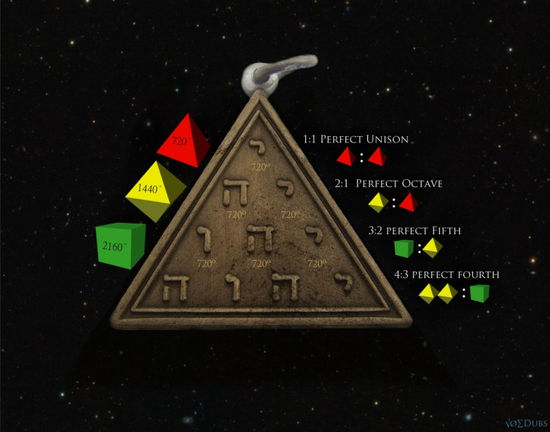top of page
Search

# Tetragrammaton=9 superstring theory

The Tetragrammaton is the name of God written in four Hebrew letters usually translated to YHWH

the tetractys and tetragrammaton encode base 10 and the decimal system and the tetractys encodes 10 dimensionsExactly ten dots are arranged to represent these perfect harmonic ratios The tetractys was venerated for its innate power in its symbolism as a perfect geometric construct for how nature organizes itself

At the heart of Pythagorean philosophy is the triangular pattern of 10 dots or points called the ‘tetractys’. it is the fourth triangular numbers These are numbers that are the sums of triangular arrays of dots, each denoting the number 1. For example, 1, 1+2=3, 1+2+3=6 and 1+2+3+4=10

There are 81 elements that make the infinite multyvers up and 3⁴=81 so 3⁴=3x3x3x3 so each 3=(4 elements) which are encoded in to metatrons cube and 81 encodes the number 9:there are exactly 81 stable elements(the number of protons in the nucleus of an atom).

81 is almost like the mother of all numbers.  All of the natural numbers spring from it’s reciprocal, as previously shown.  Divide the mother of all numbers by the largest number beneath it, and you’ll produce zero through nine.  Notice the missing “one”.80/81 = .9876543209876543209876

81=9=72=tetragrammaton=tetractys=10Dimension

(Strings,nanites)=((Virgo)=(infinite multyvers)=(-1/12))=83333=5=pentagram=11=om=136.1=(1:36+1)=1:13=1:4=13:16=4:7=28=(16(7)x4)=(64=(4³=6,6,6,6=24))=(64 tetrahedron grid=((2(tetragrammatons))=144=9)=((metatrons cube)=(flower of life)=(fruit of life))=(E8)=(xen particle)=8(tree of life's)=((crown)=(god goes to infinity))=2(star tetrahedrons=(energy))=wave=atom